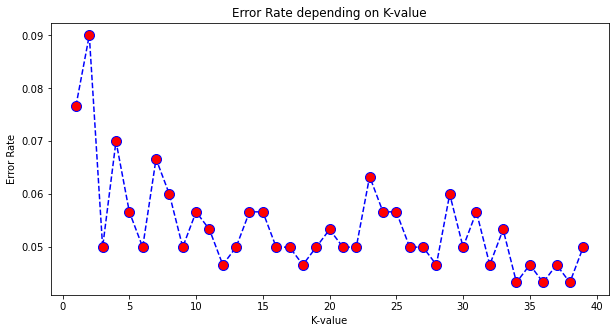Just a little example on how to use the K-Nearest Neighbor model in Python. In this example we’re utilizing an anonymous dataset with unknown context. All we know is that there is a TARGET CLASS of 0/1 and we want to predict if a row belongs to that class or not. K-Nearest Neighbor functions by comparing a point to a certain number of points around it. It’s pretty simple to implement. The dataset can be found on my github here.

## K-Nearest Neighbor - Simple Example¶

### I.E. predict value based on clustering of values surrounding a point¶

Utilizes anon data, unknown columns or context. We want to find out if a value should exist within the TARGET CLASS or not.

In :
```#Imports
import pandas as pd
import numpy as np
import matplotlib.pyplot as plt
import seaborn as sns
%matplotlib inline
```
In :
```#Read in the data
```
In :
```df.head()
```
Out:
WTT PTI EQW SBI LQE QWG FDJ PJF HQE NXJ TARGET CLASS
0 0.913917 1.162073 0.567946 0.755464 0.780862 0.352608 0.759697 0.643798 0.879422 1.231409 1
1 0.635632 1.003722 0.535342 0.825645 0.924109 0.648450 0.675334 1.013546 0.621552 1.492702 0
2 0.721360 1.201493 0.921990 0.855595 1.526629 0.720781 1.626351 1.154483 0.957877 1.285597 0
3 1.234204 1.386726 0.653046 0.825624 1.142504 0.875128 1.409708 1.380003 1.522692 1.153093 1
4 1.279491 0.949750 0.627280 0.668976 1.232537 0.703727 1.115596 0.646691 1.463812 1.419167 1

### Scale Standardization of dataset¶

Tranform data such that its distribution will have a mean value of 0, and standard deviation of 1 Essentially it it "standardizes" the dataset so that the scales of every metric are common, useful when comparing data of different units

In :
```#Import Sci-kit learn
from sklearn.preprocessing import StandardScaler
```
In :
```#Scaler Object
scaler = StandardScaler()
```
In :
```#Fit data to everything except Target Class column first (before transforming)
scaler.fit(df.drop('TARGET CLASS',axis=1))
```
Out:
`StandardScaler()`
In :
```#Scale the dataset, minus the TARGET ClASS column
scaled_features = scaler.transform(df.drop('TARGET CLASS',axis=1))
```
In :
```#Put the new scaled dataset into a dataframe
#Get all column names except TARGET CLASS (the last one)
df_feat = pd.DataFrame(scaled_features,columns=df.columns[:-1])
```
In :
```#Review "re-scaled" dataset
```
Out:
WTT PTI EQW SBI LQE QWG FDJ PJF HQE NXJ
0 -0.123542 0.185907 -0.913431 0.319629 -1.033637 -2.308375 -0.798951 -1.482368 -0.949719 -0.643314
1 -1.084836 -0.430348 -1.025313 0.625388 -0.444847 -1.152706 -1.129797 -0.202240 -1.828051 0.636759
2 -0.788702 0.339318 0.301511 0.755873 2.031693 -0.870156 2.599818 0.285707 -0.682494 -0.377850
3 0.982841 1.060193 -0.621399 0.625299 0.452820 -0.267220 1.750208 1.066491 1.241325 -1.026987
4 1.139275 -0.640392 -0.709819 -0.057175 0.822886 -0.936773 0.596782 -1.472352 1.040772 0.276510

### Split data into test/train and predict¶

In :
```#Import splitting library
from sklearn.model_selection import train_test_split
```
In :
```#Set X,Y
X = df_feat
y = df['TARGET CLASS']
```
In :
```#Choose the test size
#Test size = % of dataset allocated for testing (.3 = 30%)
#Random state = # of random splits
X_train, X_test, y_train, y_test = train_test_split(X, y, test_size=0.3, random_state=101)
```
In :
```#Import KNN classifier library
from sklearn.neighbors import KNeighborsClassifier
```
In :
```#Build model object
knn = KNeighborsClassifier(n_neighbors=1)
```
In :
```#Fit model
knn.fit(X_train,y_train)
```
Out:
`KNeighborsClassifier(n_neighbors=1)`
In :
```#Get predictions
predictions = knn.predict(X_test)
```
In :
```#See if the model worked, print reports (overall it worked very well)
from sklearn.metrics import classification_report, confusion_matrix
```
In :
```print(confusion_matrix(y_test,predictions))
```
```[[151   8]
[ 15 126]]
```
In :
```print(classification_report(y_test,predictions))
```
```              precision    recall  f1-score   support

0       0.91      0.95      0.93       159
1       0.94      0.89      0.92       141

accuracy                           0.92       300
macro avg       0.92      0.92      0.92       300
weighted avg       0.92      0.92      0.92       300

```

### Determining a better K-value¶

How exactly can we determine what K-value produces the least error rate?

In :
```#First let's see what error_rate we get by looping k-values

error_rate = [] #Empty

#Choose the max k-value to test (40 this time), start at 1
for i in range(1,40):
knn = KNeighborsClassifier(n_neighbors=i)
knn.fit(X_train,y_train)
predictions_i = knn.predict(X_test)
error_rate.append(np.mean(predictions_i != y_test)) #Avg times the prediction is NOT correct
```
In :
```#Plot out the error rates
plt.figure(figsize=(10,5))
plt.plot(range(1,40),error_rate,color='blue',linestyle='--',marker='o',markerfacecolor='red',markersize=10)
plt.title('Error Rate depending on K-value')
plt.xlabel('K-value')
plt.ylabel('Error Rate')
```
Out:
`Text(0, 0.5, 'Error Rate')`